# Equations and Inequalities

## Objective

Represent equations in the form ${ x+p=q }$ and ${px=q}$ using tape diagrams and balances.

## Common Core Standards

### Core Standards

?

• 6.EE.B.6 — Use variables to represent numbers and write expressions when solving a real-world or mathematical problem; understand that a variable can represent an unknown number, or, depending on the purpose at hand, any number in a specified set.

• 6.EE.B.7 — Solve real-world and mathematical problems by writing and solving equations of the form x + p = q and px = q for cases in which p, q and x are all nonnegative rational numbers.

## Criteria for Success

?

1. Understand that an equation represents a statement of balance between two expressions.
2. Draw tape diagrams to represent situations and equations.
3. Write equations to represent diagrams such as tape diagrams and balanced mobiles.

## Tips for Teachers

?

Students begin this unit by visually representing equations in diagrams, including familiar representations such as tape diagrams and some new ones such as balances and mobiles. This will help to support students’ understanding at a concrete level before they learn efficient ways to solve equations using inverse operations.

#### Remote Learning Guidance

If you need to adapt or shorten this lesson for remote learning, we suggest prioritizing Anchor Problem 2 (benefits from worked example). Find more guidance on adapting our math curriculum for remote learning here.

#### Fishtank Plus

• Problem Set
• Student Handout Editor
• Vocabulary Package

## Anchor Problems

?

### Problem 1

There are nine small boxes.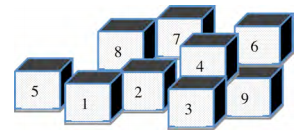They all look exactly the same, but one is a bit heavier than the others. Jake says, “I can use the scales to find the heavy one in just two steps!”

This is what Jake does first. Explain what Jake now knows about the heavy box.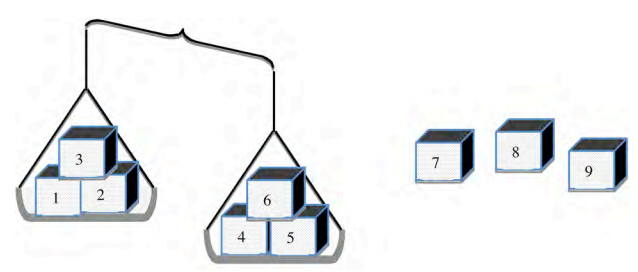Then Jake does this. Which is the heavy box? Explain how you know.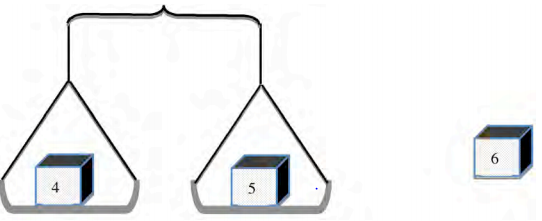#### References

Boxes of the Performance Assessment Tasks created by the by the Mathematics Assessment Resource Service (MARS) of the Shell Centre for Mathematical Education, University of Nottingham, England are made available by Inside Mathematics under a license from Shell Centre Publications. Accessed Feb. 27, 2018, 11:15 a.m..

Modified by The Match Foundation, Inc.

### Problem 2

Which tape diagram matches which situation? For each situation described, choose the tape diagram that represents it. Explain your reasoning.

Situation 1:
As party favors, Stephanie makes 5 bags and puts an equal number of pieces of candy in each bag. Stephanie uses a total of 20 pieces of candy.

Situation 2:
At a restaurant, a glass holds some water in it. The waitress comes by and adds 5 ounces of water to the glass. There are now 20 ounces of water in the glass.

Tape Diagram A: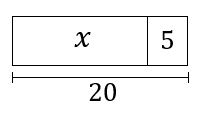Tape Diagram B: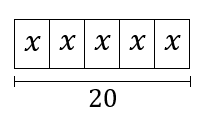### Problem 3

Show students Mobile A and Mobile B.

For each mobile shown, determine the missing value. Then write an equation to represent the situation, using the variable
${x }$ to represent the missing value.

## Problem Set

?The following resources include problems and activities aligned to the objective of the lesson that can be used to create your own problem set.

• Include problems where students draw models for equations and vice versa; include equations in the form ${x+p=q}$  and ${px=q}$
• SolveMe Mobiles (Stick to mobiles with one unknown and one tier of balances; others can be given as challenges)
• If you often use online activities to supplement instruction, we suggest the following options which match this lesson’s objective: Same thing to both sides of equations by Khan Academy and SolveMe Mobiles from the Educati on Development Center, Inc..

?

Draw a tape diagram or balance for each equation or situation below.

a.  You purchase 4 gift cards, each in the same amount. You spend a total of \$60.

b.  ${x+6=18}$

c.  ${6x=18}$

?